# 深度学习中的概率论

Posted by RAIS on 2020-03-27
• 本文首发自公众号：RAIS，期待你的关注。

## 概率论

### 概念

#### 离散型

• 概率质量函数：是一个数值，概率，$0\leq P(x)\leq 1$；
• 边缘概率分布：$P(X=x)=\sum_{y} P(X=x, Y=y)$
• 期望：$EX=\sum_xP(x)f(x)$

#### 连续型

• 概率密度函数：是一个积分，$F_X(x)=\int_{-\infty}^xf_X(t)dt$；
• 边缘概率分布：$p(x)=\int p(x,y)dy$
• 期望：$EX=\int P(x)f(x)dx$

#### 条件概率

$$P(Y=y|X=x)=\frac{P(Y=y,X=x)}{P(X=x)}$$

#### 相互独立

$$P(X=x,Y=y)=P(X=x)P(Y=y)$$

#### 条件独立

$$P(X=x,Y=y|Z=z)=P(X=x|Z=z)P(Y=y|Z=z)$$

#### 方差

$$D(X)=Var(X)=E{[X-E(X)]^2}$$

#### 标准差

$$\sigma(X)=\sqrt{D(X)}$$

#### 协方差

$$Cov(X,Y)=E{[X-E(X)][Y-E(Y)]}$$

#### 相关系数

$$\rho_{XY}=\frac{Cov(X,Y)}{\sqrt{D(X)}\sqrt{D(Y)}}$$

### 常用分布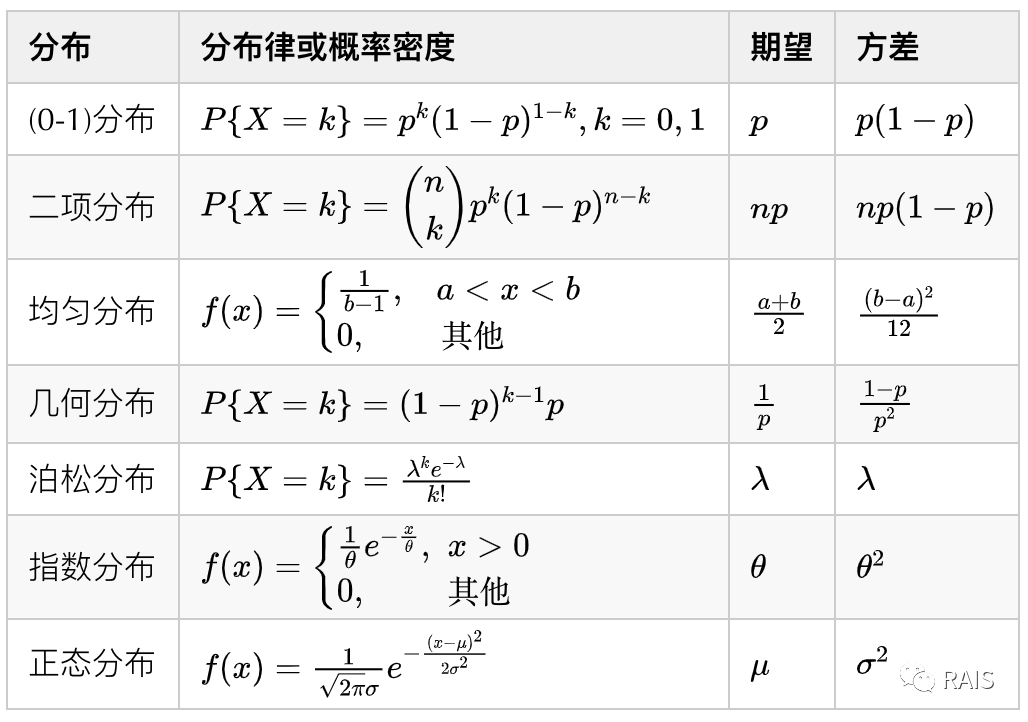#### 范畴分布（Multinoulli 分布）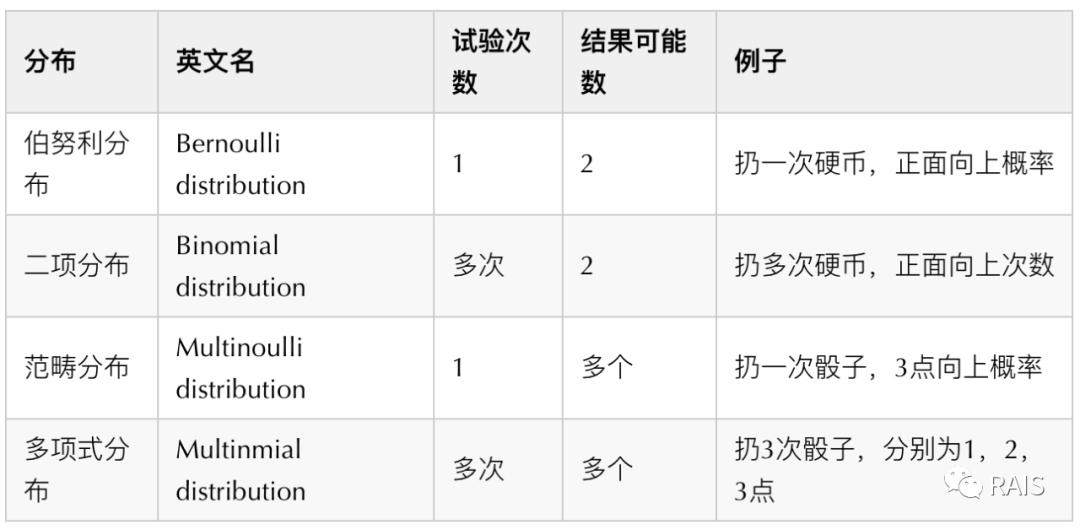#### Laplace 分布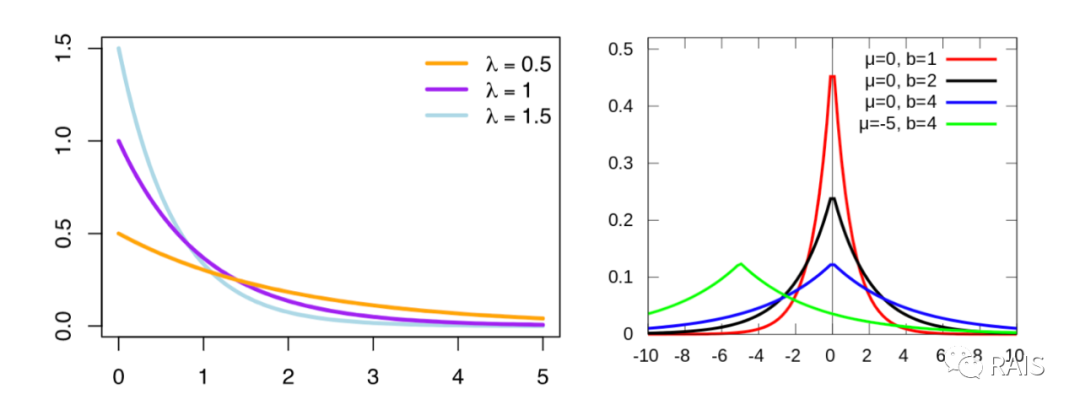#### Dirac delta 函数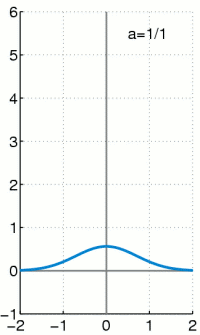#### 经验分布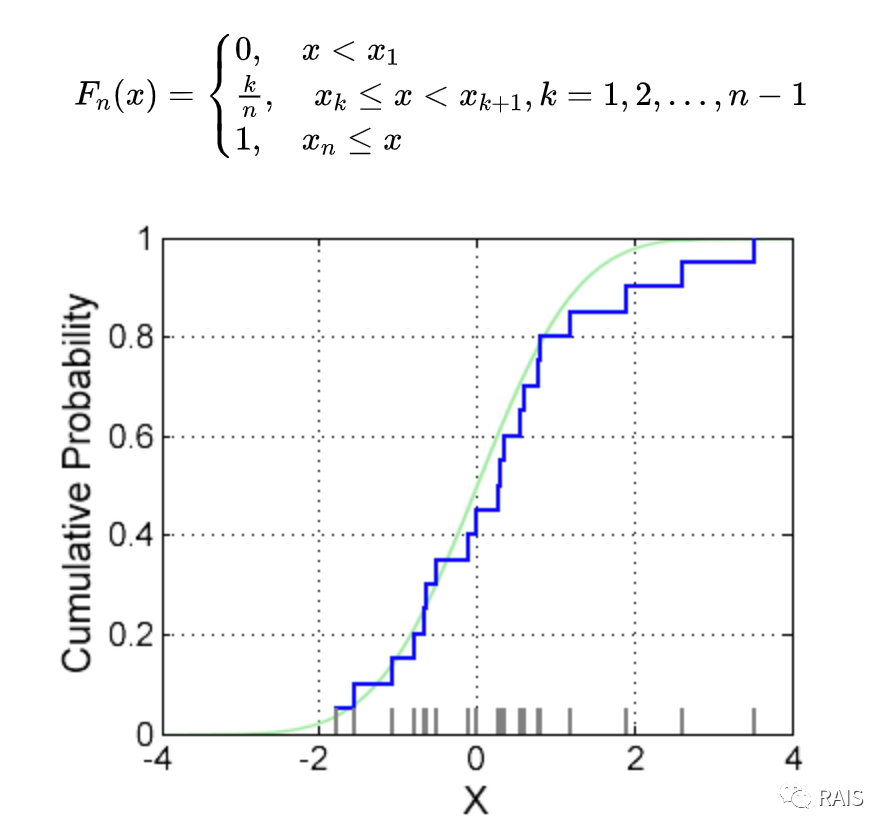### 贝叶斯规则

$$P(x|y)=\frac{P(x)P(y|x)}{P(y)}$$

## 总结

• 本文首发自公众号：RAIS，期待你的关注。